Home Practice
For learners and parents For teachers and schools
Textbooks
Full catalogue
Pricing SupportLog in

We think you are located in United States. Is this correct?

# 7.2 Equation of a circle

## 7.2 Equation of a circle (EMCHS)

### Equation of a circle with centre at the origin (EMCHT)

1. Draw a system of axes with a scale of $$\text{1}\text{ cm} = 1$$ unit on the $$x$$-axis and on the $$y$$-axis.
2. Draw the lines $$y = x$$ and $$y = -x$$.
3. Plot the following points:
1. $$O(0;0)$$
2. $$D(2;0)$$
3. $$E(\sqrt{2};\sqrt{2})$$
4. $$F(0;2)$$
5. $$G(-\sqrt{2};\sqrt{2})$$
6. $$H(-2;0)$$
7. $$I(-\sqrt{2};-\sqrt{2})$$
8. $$J(0;-2)$$
9. $$K(\sqrt{2};-\sqrt{2})$$

What object do the points form?

4. Measure the following distances and complete the table below:
 Line segment Distance $$(cm)$$ $$DO$$ $$EO$$ $$FO$$ $$GO$$ $$HO$$ $$IO$$ $$JO$$ $$KO$$
5. Use the distance formula to check the results of the above table: $\text{Distance} = \sqrt{(x_{2} - x_{1})^{2} + (y_{2} - y_{1})^2}$
 Line segment Distance $$DO$$ $$EO$$ $$FO$$ $$GO$$ $$HO$$ $$IO$$ $$JO$$ $$KO$$
6. What do you notice about the length of each line segment?
7. What is the general term given to this type of line segment?
8. If the point $$P(x;y)$$ lies on the circle, use the distance formula to determine an expression for the length of $$PO$$.
9. Can you deduce a general equation for a circle with centre at the origin?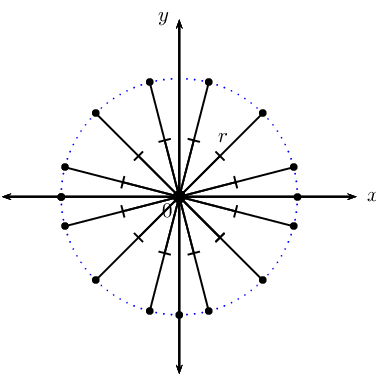A circle is the set of all points that are an equal distance (radius) from a given point (centre). In other words, every point on the circumference of a circle is equidistant from its centre.

The radius of a circle is the distance from the centre of a circle to any point on the circumference.

A diameter of a circle is any line passing through the centre of the circle which connects two points on the circle. The diameter is also the name given to the maximum distance between two points on a circle.

Consider a point $$P\left( x;y \right)$$ on the circumference of a circle of radius $$r$$ with centre at $$O\left( 0;0 \right)$$.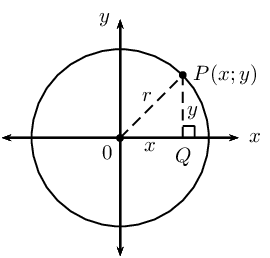In $$\triangle OPQ$$:

\begin{align*} O{P}^{2} & = P{Q}^{2}+O{Q}^{2} \quad \left( \text{Pythagoras} \right) \\ OP & = r \\ PQ & = y - 0 \\ OQ & = x - 0 \\ {r}^{2} & = {\left( y - 0\right)}^{2}+{\left( x - 0 \right)}^{2} \\ \therefore {r}^{2} & = x^{2} + y^{2} \end{align*}

Equation of a circle with centre at the origin:

If $$P(x;y)$$ is a point on a circle with centre $$O(0;0)$$ and radius $$r$$, then the equation of the circle is:

$x^{2} + y^{2} = r^{2}$

Circle symmetry

A circle with centre $$(0;0)$$ is symmetrical about the origin: for every point $$(x;y)$$ on the circumference of a circle, there is also the point $$(-x;-y)$$.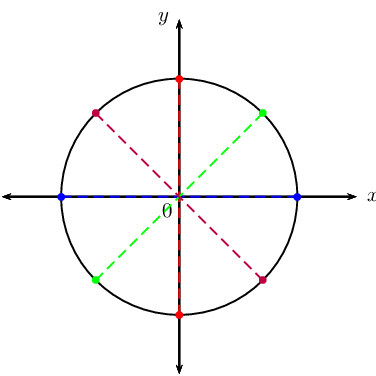A circle centred on the origin is also symmetrical about the $$x$$- and $$y$$-axis. Is a circle centred on the origin symmetrical about the lines $$y = x$$ and $$y = -x$$? How many lines of symmetry does a circle have?

## Worked example 4: Equation of a circle with centre at the origin

Given: circle with centre $$O(0;0)$$ and a radius of $$\text{3}$$ units.

1. Sketch the circle on the Cartesian plane.
2. Determine the equation of the circle.
3. Show that the point $$T \left( - \sqrt{4}; \sqrt{5} \right)$$ lies on the circle.

### Draw a sketch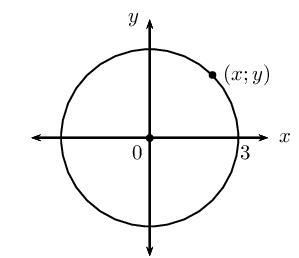### Determine the equation of the circle

Write down the general form of the equation of a circle with centre $$(0;0)$$:

\begin{align*} x^{2} + y^{2} &= r^{2} \\ \text{Substitute } r = 3: \quad x^{2} + y^{2} &= (3)^{2} \\ x^{2} + y^{2} &= 9 \end{align*}

### Show that point $$T$$ lies on the circle

Substitute the $$x$$-coordinate and the $$y$$-coordinate into the left-hand side of the equation and show that it is equal to the right-hand side:

\begin{align*} \text{LHS } &= x^{2} + y^{2} \\ &= \left( - \sqrt{4} \right)^{2} + \left( \sqrt{5} \right)^{2} \\ &= 4 + 5 \\ &= 9 \\ &= r^{2} \\ &= \text{RHS } \end{align*}

Therefore, $$T \left( - \sqrt{4}; \sqrt{5} \right)$$ lies on the circle $$x^{2} + y^{2} = 9$$.

temp text

## Worked example 5: Equation of a circle with centre at the origin

A circle with centre $$O(0;0)$$ passes through the points $$P(-5;5)$$ and $$Q(5;-5)$$.

1. Plot the points and draw a rough sketch of the circle.
2. Determine the equation of the circle.
3. Calculate the length of $$PQ$$.
4. Explain why $$PQ$$ is a diameter of the circle.

### Draw a sketch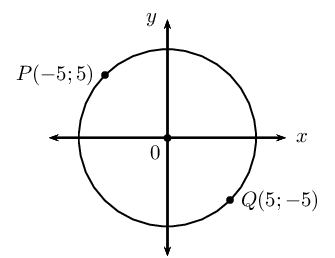### Determine the equation of the circle

Write down the general form of the equation of a circle with centre $$(0;0)$$ and substitute $$P(-5;5)$$:

\begin{align*} x^{2} + y^{2} &= r^{2} \\ (-5)^{2} + (5)^{2} &= r^{2} \\ 25 + 25 &= r^{2} \\ 50 &= r^{2} \\ \therefore r &= \sqrt{50} \quad (r \text{ is always positive}) \\ r &= 5\sqrt{2} \text{ units} \end{align*}

Therefore, the equation of the circle passing through $$P$$ and $$Q$$ is $$x^{2} + y^{2} = 50$$.

### Calculate the length $$PQ$$

Use the distance formula to determine the distance between the two points.

\begin{align*} PQ &= \sqrt{(x_{2} - x_{1})^{2} + (y_{2} - y_{1})^2} \\ &= \sqrt{(5 - (-5))^{2} + (-5 - 5)^2} \\ &= \sqrt{(10)^{2} + (-10)^2} \\ &= \sqrt{100 + 100} \\ &= \sqrt{100 \cdot 2} \\ &= 10\sqrt{2} \text{ units} \end{align*}

### Determine if $$PQ$$ is a diameter of the circle

\begin{align*} r &= 5\sqrt{2} \\ \text{And } d &= 2 \times r \\ &= 2 \times 5\sqrt{2} \\ &= 10 \sqrt{2} \\ \therefore d &= PQ \end{align*}

Since $$PQ$$ connects two points, $$P$$ and $$Q$$, on the circle and is a line of length $$10 \sqrt{2}$$ units, $$PQ$$ is a diameter of the circle.

Alternative method $$\text{1}$$: Use symmetry to show that $$PQ$$ is a diameter of the circle.

The diameter is the name given to the maximum distance between two points on a circle; this means that the two points must lie opposite each other with respect to the centre.

Using symmetry about the origin, we know that $$(x;y)$$ lies opposite $$(-x;-y)$$ on the circle and vice versa:

• $$P(-5;5)$$ lies opposite $$(5; -5)$$, which are the coordinates of $$Q$$
• $$Q(5;-5)$$ lies opposite $$(-5; 5)$$, which are the coordinates of $$P$$

Therefore, $$PQ$$ is a diameter of the circle.

Alternative method $$\text{2}$$: Show that $$PQ$$ passes through the centre.

Determine the equation of $$PQ$$ and show that it passes through the origin.

\begin{align*} \dfrac{y - y_{Q}}{x - x_{Q}} &= \dfrac{y_{P} - y_{Q}}{x_{P} - x_{Q}} \\ \dfrac{y + 5}{x - 5} &= \dfrac{5 + 5}{-5 - 5} \\ \dfrac{y + 5}{x - 5} &= \dfrac{10}{-10} \\ y + 5 &= -(x - 5) \\ y &= -x + 5 - 5 \\ y &= -x \end{align*}

$$\therefore (0;0)$$ lines on the line $$PQ$$.

Therefore, $$PQ$$ passes through the centre and is a diameter of the circle.

temp text

## Worked example 6: Equation of a circle with centre at the origin

Given a circle with centre $$O(0;0)$$ and a radius of $$\sqrt{45}$$ units. Determine the possible coordinates of the point(s) on the circle which have an $$x$$-value that is twice the $$y$$-value.

### Determine the equation of the circle

\begin{align*} x^{2} + y^{2} &= r^{2} \\ x^{2} + y^{2} &= \left( \sqrt{45} \right)^{2} \\ x^{2} + y^{2} &= 45 \end{align*}

### Determine the coordinates of the points on the circle

To calculate the possible coordinates of the point(s) on the circle which have an $$x$$-value that is twice the $$y$$-value, we substitute $$x = 2y$$ into the equation of the circle:

\begin{align*} x^{2} + y^{2} &= 45 \\ (2y)^{2} + y^{2} &= 45 \\ 4y^{2} + y^{2} &= 45 \\ 5y^{2} &= 45 \\ y^{2} &= 9 \\ \therefore y &= \pm 3 \\ \text{If } y = -3: \quad x &= 2(-3) \\ &= -6 \\ \text{If } y = 3: \quad x &= 2(3) \\ &= 6 \end{align*}

This gives the points $$(6;3)$$ and $$(-6; -3)$$.

Note: we can check that both points lie on the circle by substituting the coordinates into the equation of the circle:

\begin{align*} (6)^2 + (3)^2 &= 36 + 9 = 45 \\ (-6)^2 + (-3)^2 &= 36 + 9 = 45 \end{align*}
temp text

## Equation of a circle with centre at the origin

Textbook Exercise 7.3

Complete the following for each circle given below:

• Draw a sketch.
• Calculate the coordinates of two points on the circle.

$$x^{2} + y^{2} = 16$$

\begin{align*} x^{2} + y^{2} &= 16 \\ r^{2} &= 16 \\ \therefore r &= 4 \end{align*}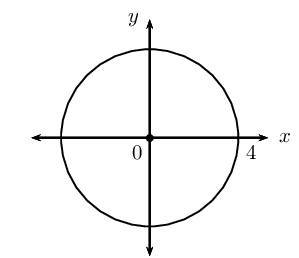This gives the points $$\left( 1; \sqrt{15} \right)$$ and $$\left( 1; -\sqrt{15} \right)$$.

$$x^{2} + y^{2} = 100$$

\begin{align*} x^{2} + y^{2} &= 100 \\ r^{2} &= 100 \\ \therefore r &= 10 \end{align*}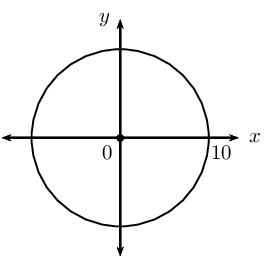This gives the points $$\left( 2; \sqrt{96} \right)$$ and $$\left( 2; -\sqrt{96} \right)$$.

$$3x^{2} + 3y^{2} = 27$$

\begin{align*} 3x^{2} + 3y^{2} &= 27 \\ x^{2} + y^{2} &= 9 \\ r^{2} &= 9 \\ \therefore r &= 3 \end{align*}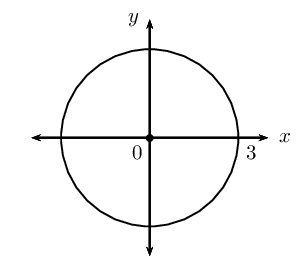This gives the points $$\left( 1; \sqrt{8} \right)$$ and $$\left( 1; -\sqrt{8} \right)$$.

$$y^{2} = 20 - x^{2}$$

\begin{align*} x^{2} + y^{2} &= 20 \\ r^{2} &= 20 \\ \therefore r &= \sqrt{20} \end{align*}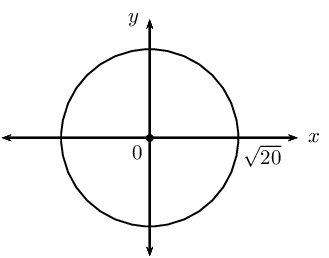This gives the points $$\left( 2; 4 \right)$$ and $$\left( 2; -4 \right)$$.

$$x^{2} + y^{2} = \text{2,25}$$

\begin{align*} x^{2} + y^{2} &= \text{2,25} \\ \text{Convert to a fraction:} \quad \text{2,25} &= \frac{225}{100} = \frac{45}{20} = \frac{9}{4} \\ \therefore x^{2} + y^{2} &= \frac{9}{4} \\ r^{2} &= \frac{9}{4} \\ \therefore r &= \frac{3}{2} \end{align*}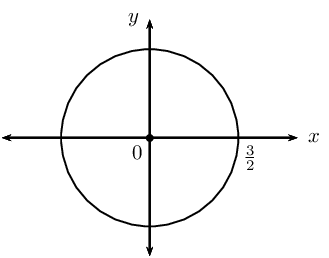This gives the points $$\left( 1; \frac{\sqrt{5}}{2} \right)$$ and $$\left( 1; -\frac{\sqrt{5}}{2} \right)$$.

$$y^{2} = - x^{2} + \frac{10}{9}$$

\begin{align*} x^{2} + y^{2} &= \frac{10}{9} \\ r^{2} &= \frac{10}{9} \\ \therefore r &= \frac{\sqrt{10}}{3} \end{align*}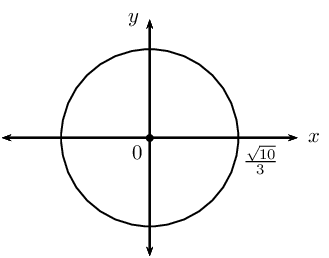This gives the points $$\left( 1; \frac{1}{3} \right)$$ and $$\left( 1; -\frac{1}{3} \right)$$.

Determine the equation of the circle:

with centre at the origin and a radius of $$\text{5}$$ units.
$$x^{2} + y^{2} = 25$$
with centre at $$(0;0)$$ and $$r = \sqrt{11}$$ units.
$$x^{2} + y^{2} = 11$$
passing through the point $$(3;5)$$ and with centre $$(0;0)$$.
\begin{align*} x^{2} + y^{2} &= r^{2} \\ (3)^{2} + (5)^{2} &= r^{2} \\ 34 &= r^{2} \\ \therefore x^{2} + y^{2} &= 34 \end{align*}
centred at the origin and $$r = \text{2,5}$$ units.
\begin{align*} x^{2} + y^{2} &= r^{2} \\ x^{2} + y^{2} &= (\text{2,5})^{2} \\ x^{2} + y^{2} &= \left( \frac{5}{2} \right)^{2} \\ x^{2} + y^{2} &= \frac{25}{4} \end{align*}
with centre at the origin and a diameter of $$\text{30}$$ units.
\begin{align*} x^{2} + y^{2} &= r^{2} \\ r &= \frac{30}{2} = 15 \\ x^{2} + y^{2} &= \left( 15 \right)^{2} \\ x^{2} + y^{2} &= 225 \end{align*}
passing through the point $$(p;3q)$$ and with centre at the origin.
\begin{align*} x^{2} + y^{2} &= r^{2} \\ (p)^{2} + (3q)^{2} &= r^{2} \\ p^{2} + 9q^{2} &= r^{2} \\ \therefore x^{2} + y^{2} &= p^{2} + 9q^{2} \end{align*}$$x^{2} + y^{2} = 1$$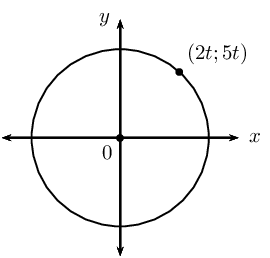\begin{align*} x^{2} + y^{2} &= r^{2} \\ (2t)^{2} + (5t)^{2} &= r^{2} \\ 4t^{2} + 25t^{2} &= r^{2} \\ 29t^{2} &= r^{2} \\ \therefore x^{2} + y^{2} &= 29t^{2} \end{align*}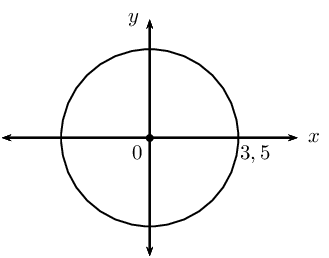\begin{align*} x^{2} + y^{2} &= r^{2} \\ x^{2} + y^{2} &= (\text{3,5})^{2} \\ x^{2} + y^{2} &= \left( \frac{7}{2} \right)^{2} \\ \therefore x^{2} + y^{2} &= \frac{49}{4} \end{align*}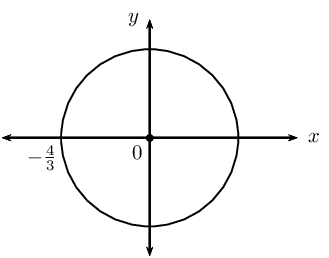\begin{align*} x^{2} + y^{2} &= r^{2} \\ x^{2} + y^{2} &= \left( -\frac{4}{3} \right)^{2} \\ \therefore x^{2} + y^{2} &= \frac{16}{9} \end{align*}

Determine whether or not the following equations represent a circle:

$$x^{2} + y^{2} - 8 = 0$$
Yes: $$x^{2} + y^{2} = 8$$
$$y^{2} - x^{2} + 25 = 0$$
No, cannot be written in the form $$x^{2} + y^{2} = r^{2}$$.
$$3x^{2} + 6y^{2} = 18$$
No, cannot be written in the form $$x^{2} + y^{2} = r^{2}$$.
$$x^{2} = \sqrt{6} - y^{2}$$
Yes: $$x^{2} + y^{2}= \sqrt{6}$$
$$y(y + x) = -x(x - y) + 11$$
Yes: $$x^{2} + y^{2}= 11$$
$$\sqrt{80} + x^{2} - y^{2} = 0$$
No, cannot be written in the form $$x^{2} + y^{2} = r^{2}$$.
$$\frac{y^{2}}{3} + \frac{x^{2}}{3} = 3$$
Yes: $$x^{2} + y^{2}= 9$$

Determine the value(s) of $$g$$ if $$\left( \sqrt{3}; g \right)$$ is a point on the circle $$x^{2} + y^{2} = 19$$.

\begin{align*} x^{2} + y^{2} &= 19 \\ \left( \sqrt{3} \right)^{2} + (g)^{2} &= 19 \\ g^{2} &= 19 - 3 \\ \therefore g^{2} &= 16 \\ \therefore g &= \pm 4 \end{align*}

This gives the points $$\left( \sqrt{3}; 4 \right)$$ and $$\left( \sqrt{3}; -4 \right)$$.

$$A(s;t)$$ is a point on the circle with centre at the origin and a diameter of $$\text{40}$$ $$\text{cm}$$.

Determine the possible coordinates of $$A$$ if the value of $$s$$ is triple the value of $$t$$.
\begin{align*} d &= 40 \\ r &= \frac{d}{2} \\ &= \frac{40}{2} \\ &= 20 \end{align*} \begin{align*} s &= 3t \\ x^{2} + y^{2} &= r^{2} \\ (3t)^{2} + (t)^{2} &= (20)^{2} \\ 9t^{2} + t^{2} &= 400 \\ 10t^{2} &= 400 \\ t^{2} &= 40 \\ \therefore t &= \pm \sqrt{40} \\ &= \pm \sqrt{4 \cdot 10} \\ &= \pm 2\sqrt{10} \\ \therefore s &= 3t \\ &= 3 \left( \pm 2\sqrt{10} \right) \\ &= \pm 6\sqrt{10} \end{align*}

Therefore, $$A \left( 6 \sqrt{10}; 2\sqrt{10} \right)$$ or $$A \left( -6 \sqrt{10}; -2\sqrt{10} \right)$$

Determine the possible coordinates of $$A$$ if the value of $$s$$ is half the value of $$t$$.
\begin{align*} d &= 40 \\ r &= \frac{d}{2} \\ &= \frac{40}{2} \\ &= 20 \end{align*} \begin{align*} s &= \frac{t}{2} \\ x^{2} + y^{2} &= r^{2} \\ \left( \frac{t}{2} \right)^{2} + (t)^{2} &= (20)^{2} \\ \frac{t^{2}}{4} + t^{2} &= 400 \\ \frac{t^{2}}{4} + \frac{4t^{2}}{4} &= 400 \\ 5t^{2} &= 1600 \\ t^{2} &= 320 \\ \therefore t &= \pm \sqrt{320} \\ &= \pm \sqrt{64 \cdot 5} \\ &= \pm 8\sqrt{5} \\ \therefore s &= \frac{t}{2} \\ &= \frac{\pm 8\sqrt{5} }{2} \\ &= \pm 4 \sqrt{5} \end{align*}

Therefore, $$A \left(4 \sqrt{5}; 8\sqrt{5} \right)$$ or $$A \left( -4 \sqrt{5}; -8\sqrt{5} \right)$$

$$P(-2;3)$$ lies on a circle with centre at $$(0;0)$$.

Determine the equation of the circle.
\begin{align*} x^{2} + y^{2} &= r^{2} \\ (-2)^{2} + (3)^{2} &= r^{2} \\ 4 + 9 &= r^{2} \\ 13 &= r^{2} \\ x^{2} + y^{2} &= 13 \end{align*}
Sketch the circle and label point $$P$$.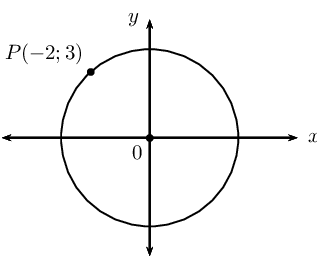If $$PQ$$ is a diameter of the circle, determine the coordinates of $$Q$$.

If $$PQ$$ is a diameter of the circle, then point $$Q$$ must lie opposite point $$P$$ on the circumference of the circle. Using symmetry about the origin, the coordinates of point $$Q$$ are $$(2;-3)$$.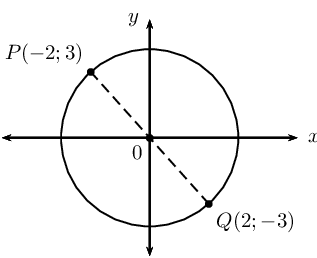Calculate the length of $$PQ$$.
\begin{align*} r^{2} &= 13 \\ \therefore r &= \sqrt{13} \\ \text{And } d &= 2 \times r \\ &= 2 \sqrt{13} \text{ units} \end{align*}

Alternative: Use the distance formula

\begin{align*} PQ &= \sqrt{(x_{2} - x_{1})^{2} + (y_{2} - y_{1})^2} \\ &= \sqrt{(-2-2)^{2} + (3-(-3))^2} \\ &= \sqrt{(-4)^{2} + (6)^2} \\ &= \sqrt{16 + 36} \\ &= \sqrt{52} \\ &= \sqrt{4 \cdot 13} \\ &= 2 \sqrt{13} \text{ units} \end{align*}
Determine the equation of the line $$PQ$$.
\begin{align*} m_{PQ} &= \frac{y_{2} - y_{1}}{x_{2}- x_{1}} \\ &= \frac{3 - (-3)}{- 2 - 2} \\ &= \frac{6}{- 4} \\ &= - \frac{3}{2} \\ y - y_{1} &= m (x - x_{1}) \\ y - y_{1} &= - \frac{3}{2} (x - x_{1}) \\ \text{Substitute } P(-2;3) \quad y - 3 &= - \frac{3}{2} (x - (-2))\\ y - 3 &= - \frac{3}{2}x - 3 \\ \therefore y &= - \frac{3}{2}x \end{align*}

$$PQ$$ passes through the origin, therefore $$c = 0$$.

Determine the equation of the line perpendicular to $$PQ$$ and passing through the point $$P$$.

For perpendicular line:

\begin{align*} m_{PQ} \times m_{\perp} &= -1 \\ - \frac{3}{2} \times m_{\perp} &= -1 \\ m_{\perp} &= \frac{2}{3} \\ y - y_{1} &= m (x - x_{1}) \\ y - y_{1} &= \frac{2}{3} (x - x_{1}) \\ \text{Substitute } P(-2;3) \quad y - 3 &= \frac{2}{3} (x - (-2)) \\ y - 3 &= \frac{2}{3}x + \frac{4}{3} \\ y &= \frac{2}{3}x + \frac{4}{3} + \frac{9}{3} \\ &= \frac{2}{3}x + \frac{13}{3} \end{align*}

## Shifting the centre of a circle

Complete the following for each equation in the table below:

• Write down the shifted equation (do not simplify).
• Draw a rough sketch to illustrate the shift(s).
1. Vertical shift: the graph is shifted $$\text{1}$$ unit up.
2. Horizontal shift: the graph is shifted $$\text{2}$$ units to the right.
3. Combined shifts: the graph is shifted $$\text{1}$$ unit up and $$\text{2}$$ units to the right.

The first example has been completed.

 Equation Vertical shift Horizontal shift Combined shift $$y - 3x^{2} = 0$$ $$(y - 1) - 3x^{2} = 0$$ $$y - 3(x - 2)^{2} = 0$$ $$(y - 1) - 3(x - 2)^{2} = 0$$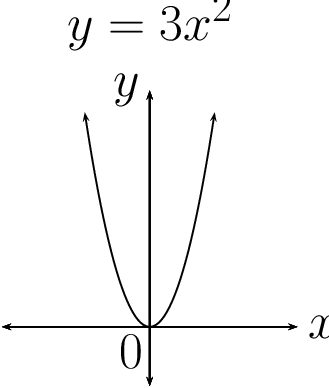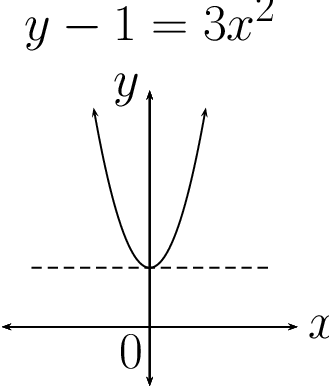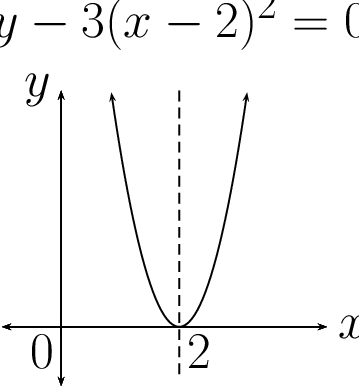$$y - 5^{x} = 0$$ $$x^{2} + y^{2} = 4$$

Use the table to answer the following questions:

1. Write down the general equation of a circle with centre $$(0;0)$$.
2. Write down the general equation of a circle with centre $$(0;b)$$.
3. Write down the general equation of a circle with centre $$(a;0)$$.
4. Write down the general equation of a circle with centre $$(a;b)$$.Consider a circle in the Cartesian plane with centre at $$C(x_{1}; y_{1})$$ and with a radius of $$r$$ units. If $$P(x_{2}; y_{2})$$ is any point on the circumference of the circle, we can use the distance formula to calculate the distance between the two points:

$PC = \sqrt{(x_{2} - x_{1})^{2} + (y_{2} - y_{1})^2}$

The distance $$PC$$ is equal to the radius ($$r$$) of the circle:

\begin{align*} r &= \sqrt{(x_{2} - x_{1})^{2} + (y_{2} - y_{1})^2} \\ \therefore r^{2} &= (x_{2} - x_{1})^{2} + (y_{2} - y_{1})^2 \end{align*}

If the coordinates of the centre of the circle are $$(a;b)$$, then the equation of a circle not centred on the origin is:

$(x_{2} - a)^{2} + (y_{2} - b)^2 = r^{2}$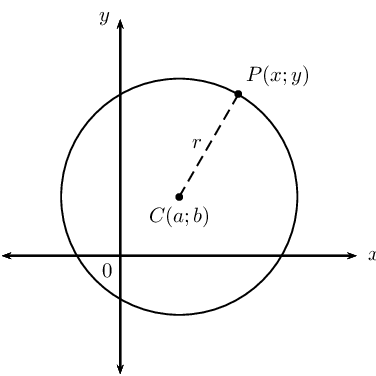Equation of a circle with centre at $$(a;b)$$:

If $$P(x;y)$$ is a point on a circle with centre $$C(a;b)$$ and radius $$r$$, then the equation of the circle is:

$(x - a)^{2} + (y - b)^{2} = r^{2}$

A circle with centre $$(0;0)$$ is a special case of the general equation:

$(x - a)^{2} + (y - b)^{2} = r^{2}$ \begin{align*} (x - 0)^{2} + (y - 0)^{2} &= r^{2} \\ \therefore x^{2} + y^{2} &= r^{2} \end{align*}

## Worked example 7: Equation of a circle with centre at $$(a;b)$$

$$F(6;-4)$$ is a point on the circle with centre $$(3;-4)$$.

1. Draw a rough sketch of the circle and label $$F$$.
2. Determine the equation of the circle.
3. Does the point $$G \left( \frac{3}{2} ; -2 \right)$$ lie on the circle?
4. Does the circle cut the $$y$$-axis? Motivate your answer.

### Draw a sketch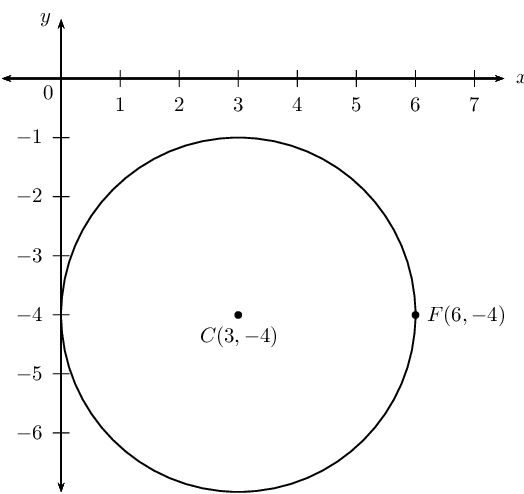### Determine the equation of the circle

Write down the general equation of a circle with centre $$(a;b)$$ and substitute the coordinates $$(3;-4)$$:

\begin{align*} (x - a)^{2} + (y - b)^{2} &= r^{2} \\ (x - 3)^{2} + (y - (-4))^{2} &= r^{2} \\ (x - 3)^{2} + (y + 4)^{2} &= r^{2} \end{align*}

Substitute the coordinates of $$F(6;-4)$$ to determine the value of $$r^{2}$$:

\begin{align*} (6 - 3)^{2} + (-4 + 4)^{2} &= r^{2} \\ (3)^{2} + (0)^{2} &= r^{2} \\ 9 &= r^{2} \\ \therefore (x - 3)^{2} + (y + 4)^{2} &= 9 \end{align*}

### Determine whether or not $$G$$ lies on the circle

If $$G \left( \frac{3}{2} ; -2 \right)$$ lies on the circle, then it will satisfy the equation of the circle:

\begin{align*} \text{LHS } &= \left( \frac{3}{2} - 3 \right)^{2} + \left( -2 + 4 \right)^{2} \\ &= \left( - \frac{3}{2} \right)^{2} + \left( 2 \right)^{2} \\ &= \frac{9}{4} + 4 \\ &= \frac{9}{4} + \frac{16}{4} \\ &= \frac{25}{4} \end{align*}\begin{align*} \text{RHS } &= 9 \\ \therefore \text{LHS } &\ne \text{ RHS} \end{align*}

Therefore $$G$$ does not lie on the circle.

### Determine the $$y$$-intercept(s)

To determine the $$y$$-intercept(s), we let $$x = 0$$:

\begin{align*} (0 - 3)^{2} + (y + 4)^{2} &= 9 \\ 9 + (y + 4)^{2} &= 9 \\ (y + 4)^{2} &= 0 \\ y + 4 &= 0 \\ \therefore y &= -4 \end{align*}

The circle cuts the $$y$$-axis at $$(0;-4)$$.

temp text

## Worked example 8: Equation of a circle with centre at $$(a;b)$$

Determine the coordinates of the centre of the circle and the length of the radius for $$3x^{2} + 6x + 3y^{2} - 12y - 33 = 0$$.

### Make the coefficient of the $$x^{2}$$ term and the $$y^{2}$$ term equal to $$\text{1}$$

The coefficient of the $$x^{2}$$ and $$y^{2}$$ term must be $$\text{1}$$, so we take out $$\text{3}$$ as a common factor:

$x^{2} + 2x + y^{2} - 4y - 11 = 0$

### Complete the square

Take half the coefficient of the $$x$$ term, square it; then add and subtract it from the equation.

The coefficient of the $$x$$ term is $$\text{2}$$, so then $$\left( \frac{2}{2} \right)^{2} = (1)^{2} = 1$$.

Take half the coefficient of the $$y$$ term, square it; then add and subtract it from the equation.

The coefficient of the $$y$$ term is $$-\text{4}$$, so then $$\left( \frac{-4}{2} \right)^{2} = (-2)^{2} = 4$$.

\begin{align*} x^{2} + 2x + y^{2} - 4y - 11 &= 0 \\ (x^{2} + 2x + 1 ) - 1 + (y^{2} - 4y + 4) -4 - 11 &= 0 \\ \left( x + 1 \right)^{2} + \left( y - 2 \right)^{2} - 16 &= 0 \\ \left( x + 1 \right)^{2} + \left( y - 2 \right)^{2} &= 16 \end{align*}

The centre of the circle is $$(-1;2)$$ and the radius is $$\text{4}$$ units.

temp text

## Worked example 9: Equation of a circle with centre at $$(a;b)$$

Given $$S(-3;4)$$ and $$T(-3;-4)$$ on the Cartesian plane.1. The points $$U$$ and $$V$$ are symmetrical about the origin to $$S$$ and $$T$$ respectively. Determine the coordinates of $$U$$ and $$V$$.
2. Determine the mid-point of $$SU$$.
3. Write down the equation of the circle $$STUV$$.
4. Is $$S\hat{T}U = \text{90} °$$? Give reasons.
5. Determine the equation of the line perpendicular to $$SU$$ and passing through point $$S$$.

### Determine the coordinates of $$U$$ and $$V$$

For symmetry about the origin, every point $$(x; y)$$ is symmetrical to $$(−x; −y)$$. So $$S(-3;4)$$ is symmetrical to $$U(3;-4)$$ and $$T(-3;-4)$$ is symmetrical to $$V(3;4)$$.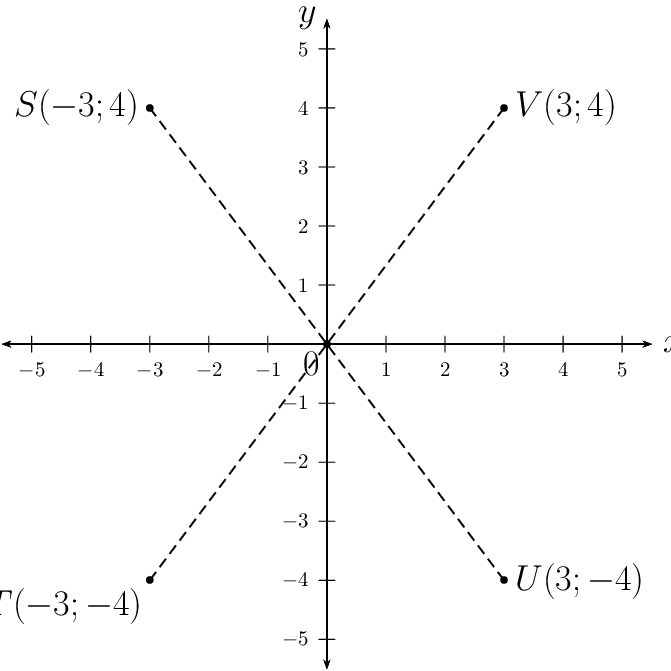### Determine the mid-point of $$SU$$

\begin{align*} M(x;y) &= \left( \frac{x_{U} + x_{S}}{2}; \frac{y_{U} + y_{S}}{2} \right) \\ &= \left( \frac{3 - 3}{2}; \frac{- 4 + 4}{2} \right) \\ &= \left( 0; 0 \right) \end{align*}

The mid-point of the line $$SU$$ is the origin.

### Determine the equation of the circle

\begin{align*} x^{2} + y^{2} &= r^{2} \\ (-3)^{2} + (4)^{2} &= r^{2} \\ 9 + 16 &= r^{2} \\ 25 &= r^{2} \\ \therefore x^{2} + y^{2} &= 25 \end{align*}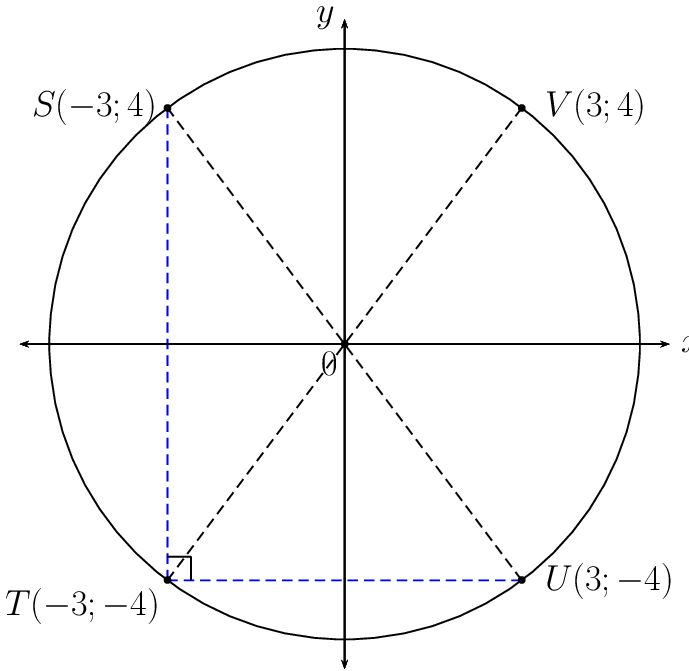$$SU$$ passes through the centre of the circle and is therefore a diameter. From Euclidean geometry, we know that the diameter of the circle subtends a right-angle at the circumference, therefore $$S\hat{T}U = \text{90} °$$ (angle in semi-circle).

### Determine the equation of the line perpendicular to $$SU$$ at point $$S$$

Determine the gradient of $$SU$$:

\begin{align*} m_{SU} &= \frac{y_{S} - y_{U}}{x_{S}- x_{U}} \\ &= \frac{4 - (- 4)}{-3 - 3} \\ &= \frac{8}{-6} \\ &= - \frac{4}{3} \end{align*}

Let the gradient of the line perpendicular to $$SU$$ be $$m_{P}$$:

\begin{align*} m_{SU} \times m_{P} &= -1 \\ - \frac{4}{3} \times m_{P} &= -1 \\ \therefore m_{P} &= \frac{3}{4} \\ y - y_{1} &= m(x - x_{1}) \\ \text{Substitute } S(-3;4): \quad y - 4 &= \frac{3}{4} (x - (-3)) \\ y - 4 &= \frac{3}{4} (x + 3) \\ y &= \frac{3}{4}x + \frac{25}{4} \end{align*}

## Worked example 10: Equation of a circle with centre at $$(a;b)$$

Given: a circle with centre $$(0;0)$$ and a radius of $$\text{4}$$ units.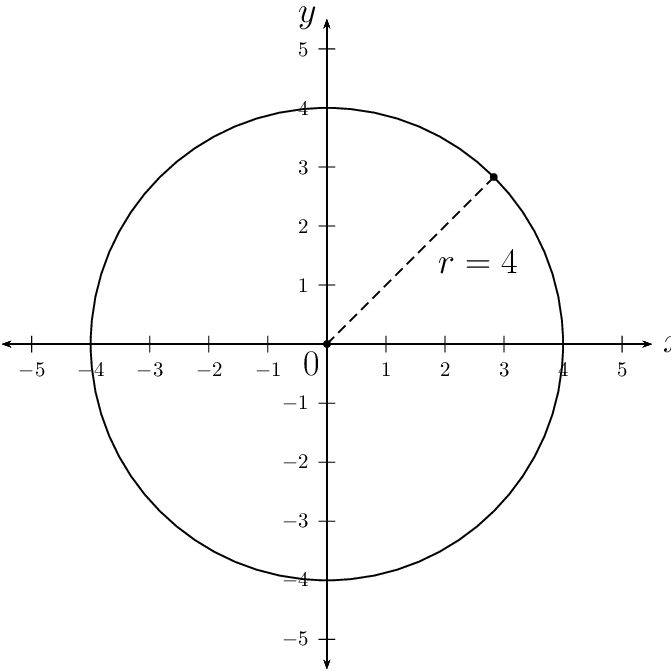1. If the circle is shifted $$\text{2}$$ units down and $$1$$ unit to the right, write down the equation of the shifted circle.
2. Sketch the original circle and the shifted circle on the same system of axes.
3. The shifted circle is reflected about the line $$y = x$$. Sketch the reflected circle on the same system of axes as the question above.
4. Write down the equation of the reflected circle.

### Write down the equation of the circle

$x^{2} + y^{2} = 16$

### Determine the equation of the shifted circle

• Vertical shift: $$\text{2}$$ units down: $$y$$ is replaced with $$y + 2$$
• Horizontal shift: $$\text{1}$$ unit to the right: $$x$$ is replaced with $$x - 1$$

Therefore the equation of the shifted circle is $$(x - 1)^{2} + (y + 2)^{2} = 16$$ with centre at $$(1;-2)$$ and radius of $$4$$ units.

### Draw a sketch of the circles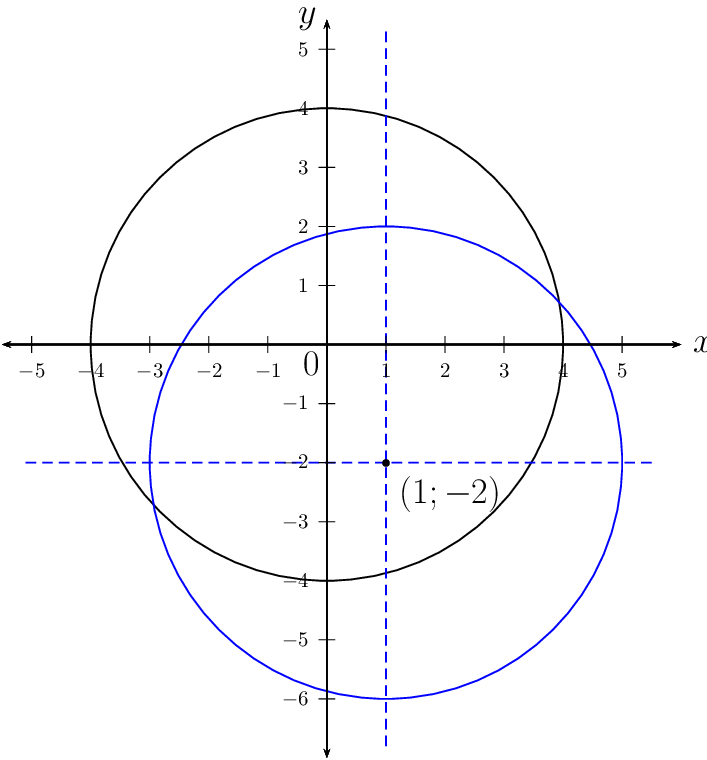The shifted circle is reflected about the line $$y = x$$. The $$x$$ and $$y$$ variables are interchanged to give the circle with equation $$(y - 1)^{2} + (x + 2)^{2} = 16$$ and centre at $$(-2;1)$$.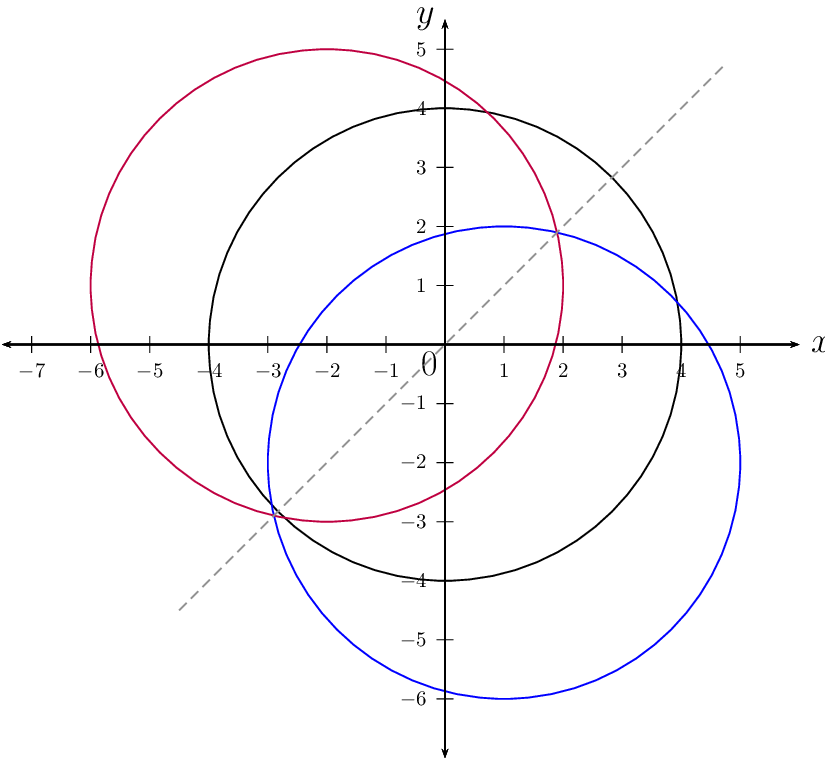## Worked example 11: Equation of a circle with centre at $$(a;b)$$

A circle with centre on the line $$y = -x + 5$$ passes through the points $$P(5;8)$$ and $$Q(9;4)$$. Determine the equation of the circle.

### Draw a rough sketch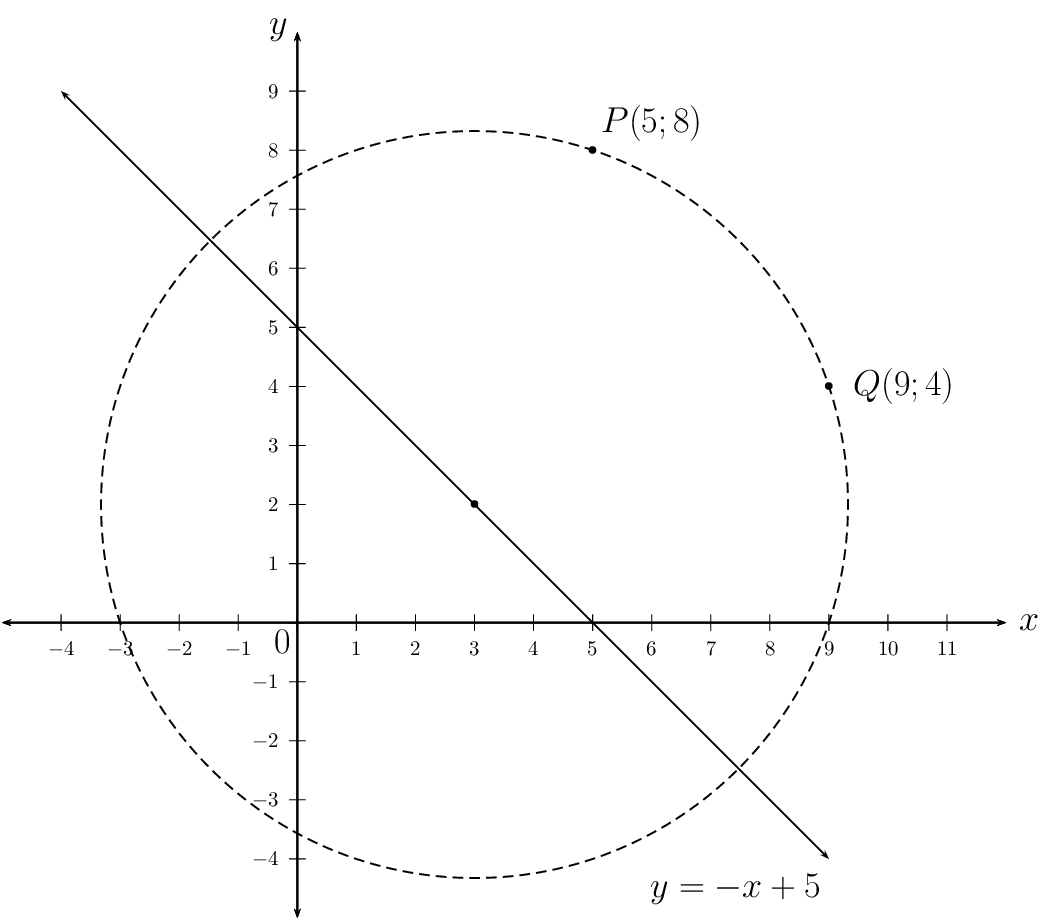### Write down the general equation of a circle

$(x - a)^{2} + (y - b)^{2} = r^{2}$

Consider the line $$y = -x + 5$$. Any point on this line will have the coordinates $$(x; - x + 5)$$. Since the centre of the circle lies on the line $$y = -x + 5$$, we can write the equation of the circle as

\begin{align*} (x - a)^{2} + (y - (- a + 5))^{2} & = r^{2} \\ (x - a)^{2} + (y + a - 5)^{2} & = r^{2} \end{align*}

### Solve for the unknown variables $$a$$ and $$r$$

We need two equations to solve for the two unknown variables. We substitute the two given points, $$P(5;8)$$ and $$Q(9;4)$$ and solve for $$a$$ and $$r$$ simultaneously:

\begin{align*} \text{Substitute } P(5;8): \quad (5 - a)^{2} + (8 + a - 5)^{2} & = r^{2} \\ (5 - a)^{2} + (a + 3)^{2} & = r^{2} \\ 25 - 10a + a^{2} + a^{2} + 6a + 9 & = r^{2} \\ 2a^{2} - 4a + 34 & = r^{2} \ldots (1) \\ & \\ \text{Substitute } Q(9;4): \quad (9 - a)^{2} + (4 + a - 5)^{2} & = r^{2} \\ (9 - a)^{2} + (a - 1)^{2} & = r^{2} \\ 81 - 18a + a^{2} + a^{2} - 2a + 1 & = r^{2} \\ 2a^{2} - 20a + 82 & = r^{2} \ldots (2) \\ & \\ (1) - (2): \quad 16a - 48 &= 0 \\ 16a &= 48 \\ \therefore a &= 3 \\ \text{Substitute into } (2): \quad r^{2} &= 2(3)^{2} - 20 (3) + 82 \\ &= 18 - 60 + 82 \\ &= 40 \\ \text{And } \quad b &= -a + 5 \\ &= -3 + 5 \\ &= 2 \end{align*}

The equation of the circle is $$(x - 3)^{2} + (y - 2)^{2} = 40$$.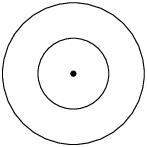Circles with the same centre.Circles touch internally at one point: the distance between the centres is equal to the difference between the radii. $$d = r_{2} - r_{1}$$Circles intersect at two points: the distance between the centres is less than the sum of the radii. $$d < r_{1} + r_{2}$$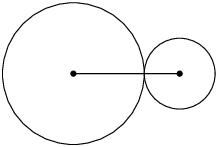Circles touch externally at one point: the distance between the centres is equal to the sum of the radii. $$d = r_{1} + r_{2}$$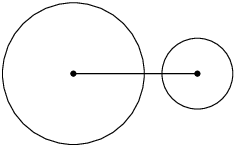Circles do not touch or intersect: the distance between the centres is greater than the sum of the radii. $$d > r_{1} + r_{2}$$

## Equation of a circle with centre at $$(a;b)$$

Textbook Exercise 7.4

Determine whether or not each of the following equations represents a circle. If not, give a reason.

$$x^{2} + y^{2} + 6y - 10 = 0$$
Yes
$$3x^{2} - 35 + 3y^{2} = 9y$$
Yes
$$40 = x^{2} + 2x + 4y^{2}$$
No, coefficients of $$x^{2}$$ term and $$y^{2}$$ term are different.
$$x^{2} - 4x = \sqrt{21} + 5y + y^{2}$$
No, cannot be written in general form $$(x - a)^{2} + (y - b)^{2} = r^{2}$$
$$3\sqrt{7} - x^{2} - y^{2} + 6y - 8x = 0$$
Yes
$$(x - 1)^{2} + (y + 2)^{2} + 9 = 0$$
No, $$r^{2}$$ must be greater than zero.

Write down the equation of the circle:

with centre $$(0;4)$$ and a radius of $$\text{3}$$ units.
$$x^{2} + (y - 4)^{2} = 9$$
such that $$r = 5$$ and the centre is the origin.
$$x^{2} + y^{2} = 25$$
with centre $$(-2;3)$$ and passing through the point $$(4;5)$$.
\begin{align*} (x - a)^{2} + (y - b)^{2} &= r^{2} \\ (x - (-2))^{2} + (y - 3)^{2} &= r^{2} \\ (x + 2)^{2} + (y - 3)^{2} &= r^{2} \\ \text{Substitute } (4;5): \quad (4 + 2)^{2} + (5 - 3)^{2} &= r^{2} \\ (6)^{2} + (2)^{2} &= r^{2} \\ 36 + 4 &= r^{2} \\ 40 &= r^{2} \\ \therefore (x + 2)^{2} + (y - 3)^{2} &= 40 \end{align*}
with centre $$(p;-q)$$ and $$r = \sqrt{6}$$.
$$(x - p)^{2} + (y + q)^{2} = 6$$
with $$r = \sqrt{10}$$ and centre $$\left( -\frac{1}{2}; \frac{3}{2} \right)$$ and
$$\left( x + \frac{1}{2} \right)^{2} + \left( y - \frac{3}{2} \right)^{2} = 10$$
with centre $$(1;-5)$$ and passing through the origin.
\begin{align*} (x - a)^{2} + (y - b)^{2} &= r^{2} \\ (x - 1)^{2} + (y - (-5))^{2} &= r^{2} \\ (x - 1)^{2} + (y + 5)^{2} &= r^{2} \\ \text{Substitute } (0;0): \quad (0 - 1)^{2} + (0 + 5)^{2} &= r^{2} \\ 1 + 25 &= r^{2} \\ 26 &= r^{2} \\ \therefore (x - 1)^{2} + (y + 5)^{2} &= 26 \end{align*}

Determine the centre and the length of the radius for the following circles:

$$x^{2} = 21 - y^{2} + 4y$$
\begin{align*} x^{2} &= 21 - y^{2} + 4y \\ x^{2} + y^{2} - 4y &= 21 \\ x^{2} + (y^{2} - 4y + 4) - 4 &= 21 \\ x^{2} + (y - 2)^{2} &= 25 \end{align*}

Centre: $$(0;2)$$, $$r = 5$$ units

$$y^{2} + x + x^{2} - \frac{15}{4} = 0$$
\begin{align*} y^{2} + x + x^{2} - \frac{15}{4} &= 0 \\ x^{2} + x + y^{2} &= \frac{15}{4} \\ \left( x^{2} + x + \frac{1}{4} \right) - \frac{1}{4} + y^{2} &= \frac{15}{4} \\ \left( x + \frac{1}{2} \right)^{2} + y^{2} &= \frac{16}{4} \\ \left( x + \frac{1}{2} \right)^{2} + y^{2} &= 4 \end{align*}

Centre: $$\left(-\frac{1}{2}; 0 \right)$$, $$r = 2$$ units

$$x^{2} - 4x + y^{2} + 2y - 5 = 0$$
\begin{align*} x^{2} - 4x + y^{2} + 2y - 5 &= 0 \\ (x^{2} - 4x + 4) - 4 + (y^{2} + 2y + 1) - 1 - 5 &= 0 \\ (x - 2)^{2} + (y + 1)^{2} - 10 &= 0 \\ (x - 2)^{2} + (y + 1)^{2} &= 10 \end{align*}

Centre: $$(2;-1)$$, $$r = \sqrt{10}$$ units

$$x^{2} + y^{2} - 6y + 2x - 15 = 0$$
\begin{align*} x^{2} + 2x + y^{2} - 6y &= 15 \\ (x^{2} + 2x + 1) - 1 + (y^{2} - 6y + 9) - 9 &= 15 \\ (x + 1)^{2} + (y - 3)^{2} &= 25 \end{align*}

Centre: $$(-1;3)$$, $$r = 5$$ units

$$5 - x^{2} - 6x - 8y - y^{2} = 0$$
\begin{align*} 5 - x^{2} - 6x - 8y - y^{2} &= \\ x^{2} + 6x + y^{2} + 8y &= 5 \\ (x^{2} + 6x + 9) - 9 + (y^{2} + 8y + 16) - 16 &= 5 \\ (x + 3)^{2} + (y + 4)^{2} &= 30 \end{align*}

Centre: $$(-3;-4 )$$, $$r = \sqrt{30}$$ units

$$x^{2} - \frac{2}{3}x + y^{2} - 4y = \frac{35}{9}$$
\begin{align*} x^{2} - \frac{2}{3}x + y^{2} - 4y &= \frac{35}{9} \\ \left( x^{2} - \frac{2}{3}x + \frac{1}{9} \right) - \frac{1}{9} + (y^{2} - 4y + 4) - 4 &= \frac{35}{9} \\ \left( x - \frac{1}{3} \right)^{2} + (y - 2)^{2} &= \frac{35}{9} + \frac{1}{9} + 4 \\ \left( x - \frac{1}{3} \right)^{2} + (y - 2)^{2} &= 8 \end{align*}

Centre: $$\left( \frac{1}{3}; 2\right)$$, $$r = \sqrt{8}$$ units

$$16x + 2y^{2} - 20y + 2x^{2} + 42 = 0$$
\begin{align*} 16x + 2y^{2} - 20y + 2x^{2} + 42 &= 0 \\ 2x^{2} + 16x + 2y^{2} -20y &= -42 \\ x^{2} + 8x + y^{2} - 10y &= -21 \\ (x^{2} + 8x + 16) - 16 + (y^{2} - 10y + 25) - 25 &= -21 \\ (x + 4)^{2} + (y - 5)^{2} &= 20 \end{align*}

Centre: $$(-4;5 )$$, $$r = \sqrt{20}$$ units

$$6x - 6y - x^{2} - y^{2} = 6$$
\begin{align*} 6x - 6y - x^{2} - y^{2} &= 6 \\ x^{2} - 6x + y^{2} + 6y &= -6 \\ (x^{2} - 6x + 9) - 9 + (y^{2} + 6y + 9) - 9 &= -6 \\ (x - 3)^{2} + (y + 3)^{2} &= 12 \end{align*}

Centre: $$(3;-3)$$, $$r = \sqrt{12}$$ units

A circle cuts the $$x$$-axis at $$R(-2;0)$$ and $$S(2;0)$$. If $$r = \sqrt{20}$$ units, determine the possible equation(s) of the circle. Draw a sketch.

\begin{align*} (x - a)^{2} + (y - b)^{2} &= r^{2} \\ (x - a)^{2} + (y - b)^{2} &= 20 \\ \text{Substitute } R(-2;0): \quad (-2 - a)^{2} + (0 - b)^{2} &= 20 \\ 4 + 4a + a^{2} + b^{2} &= 20 \\ 4a + a^{2} + b^{2} &= 16 \ldots (1) \\ \text{Substitute } S(2;0): \quad (2 - a)^{2} + (0 - b)^{2} &= 20 \\ 4 - 4a + a^{2} + b^{2} &= 20 \\ - 4a + a^{2} + b^{2} &= 16 \ldots (2) \\ (1) - (2): \quad 4a - (-4a) &= 0 \\ 8a &= 0 \\ \therefore a &= 0 \\ \text{And } b^{2} &= 16 \\ \therefore b &= \pm 4 \end{align*}

The equation of the circle passing through points $$R$$ and $$S$$ is $$x^{2} + (y + 4)^{2} = 20$$ or $$x^{2} + (y - 4)^{2} = 20$$.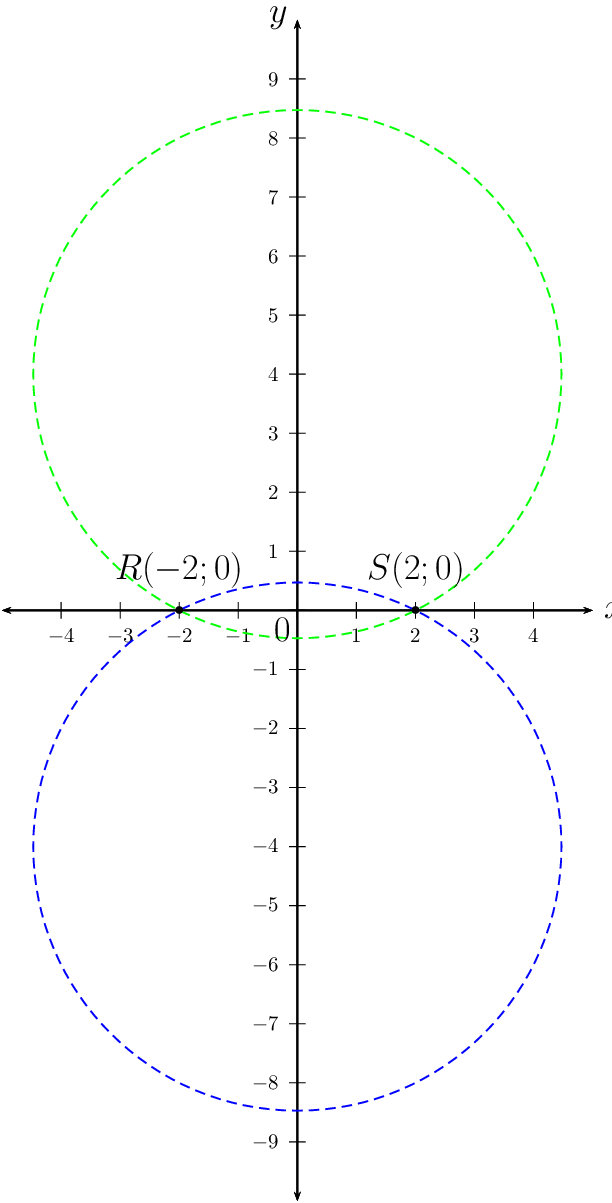$$P(1;2)$$ and $$Q(-5;-6)$$ are points on a circle such that $$PQ$$ is a diameter. Determine the equation of the circle.

Use the distance formula to determine the length of the diameter.

\begin{align*} PQ &= \sqrt{ (x_{2} - x_{1})^{2} + (y_{2} - y_{1})^{2} } \\ &= \sqrt{ (- 5 - 1)^{2} + (-6 - 2)^{2} } \\ &= \sqrt{ (- 6)^{2} + (-8)^{2} } \\ &= \sqrt{36 +64} \\ &= \sqrt{100} \\ &= 10 \\ \text{And } r &= \frac{1}{2} \times \text{ diameter} \\ &= \frac{1}{2} \times 10 \\ &= 5 \\ (x - a)^{2} + (y - b)^{2} &= (5)^{2} \\ (x - a)^{2} + (y - b)^{2} &= 25 \end{align*}

Given $$PQ$$ is a diameter of the circle, then the centre is the mid-point of PG:

\begin{align*} M(x;y) &= \left( \frac{x_{1} + x_{2}}{2}; \frac{y_{1} + y_{2}}{2} \right) \\ &= \left( \frac{1 - 5}{2}; \frac{2 - 6}{2} \right) \\ &= \left( \frac{-4}{2}; \frac{-4}{2} \right) \\ &= \left(-2; -2 \right) \end{align*}

Therefore the centre is $$(-2;-2)$$ and the equation of the circle is $$(x + 2)^{2} + (y + 2)^{2} = 25$$.

A circle with centre $$N(4;4)$$ passes through the points $$K(1;6)$$ and $$L(6;7)$$.

Determine the equation of the circle.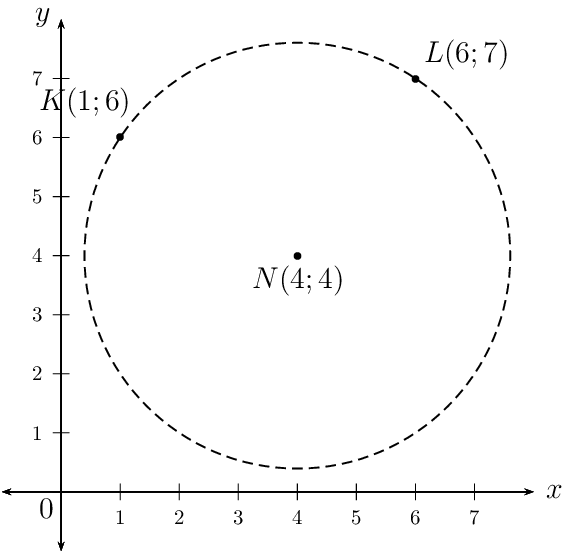The equation of the circle is $$(x - 4)^{2} + (y - 4)^{2} = 13$$ .

Determine the coordinates of $$M$$, the mid-point of $$KL$$.
\begin{align*} M(x;y) &= \left( \frac{x_{1} + x_{2}}{2}; \frac{y_{1} + y_{2}}{2} \right) \\ &= \left( \frac{1 + 6}{2}; \frac{6 + 7}{2} \right) \\ &= \left( \frac{7}{2}; \frac{13}{2} \right) \end{align*}
Show that $$MN \perp KL$$.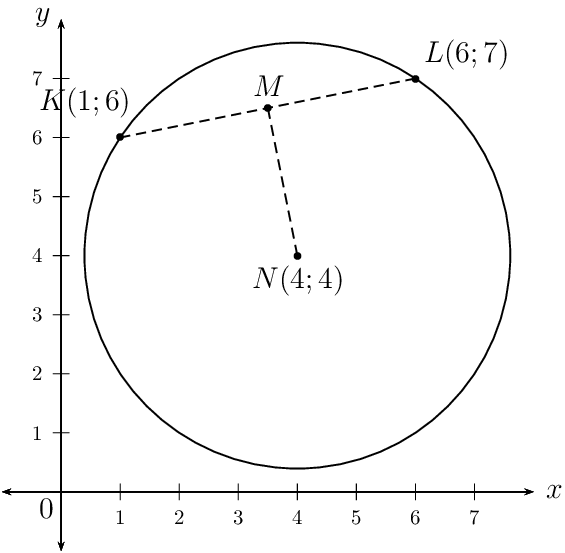If $$P$$ is a point on the circle such that $$LP$$ is a diameter, determine the coordinates of $$P$$.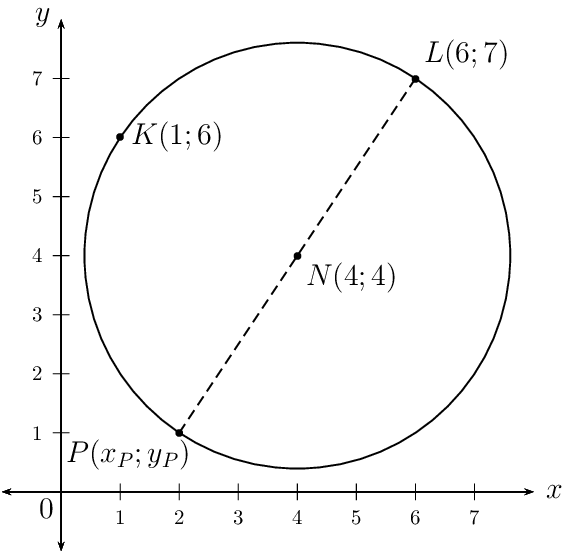Use the mid-point formula to calculate the coordinates of $$P$$:

\begin{align*} (x;y) = \left( \frac{x_{1} + x_{2}}{2}; \frac{y_{1} + y_{2}}{2} \right) \\ N(4;4) = \left( \frac{x_{P} + 6}{2}; \frac{y_{P} + 7}{2} \right) \\ \therefore 4 &= \frac{x_{P} + 6}{2} \\ 8 &= x_{P} + 6 \\ x_{P} &= 2 \\ \text{And } 4 &= \frac{y_{P} + 7}{2} \\ 8 &= y_{P} + 7\\ y_{P} &= 1 \\ \therefore & P(2;1) \end{align*}
Determine the equation of the line $$LP$$.
\begin{align*} m_{LP} &= \frac{y_{2} - y_{1}}{x_{2}- x_{1}} \\ &= \frac{7 - 1}{6 - 2 } \\ &= \frac{6}{4} \\ &=\frac{3}{2} \\ y - y_{1} &= m(x - x_{1}) \\ y - y_{1} &=\frac{3}{2}(x - x_{1}) \\ \text{Substitute } L(6;7) y -7 &=\frac{3}{2}(x - 6) \\ y &=\frac{3}{2}x - 9 + 7 \\ y &=\frac{3}{2}x - 2 \end{align*}
A circle passes through the point $$A(7;-4)$$ and $$B(-5;-2)$$. If its centre lies on the line $$y + 5 = 2x$$, determine the equation of the circle.

Given that the centre of the circle lies on the line $$y = 2x - 5$$, we can write the coordinates of the circle as $$(p; 2p - 5)$$ and the equation of the circle becomes:

\begin{align*} (x - a)^{2} + (y - b)^{2} &= r^{2} \\ (x - p)^{2} + (y - (2p - 5))^{2} &= r^{2} \\ (x - p)^{2} + (y - 2p + 5)^{2} &= r^{2} \\ \text{Substitute } A(7;4): \quad (7 - p)^{2} + (-4 - 2p + 5)^{2} &= r^{2} \\ (7 - p)^{2} + (1 - 2p)^{2} &= r^{2} \\ 49 - 14p + p^{2} + 1 -4p + 4p^{2} &= r^{2} \\ 5p^{2} - 18p + 50 &= r^{2} \ldots (1) \\ \text{Substitute } B(-5;-2): \quad (-5 - p)^{2} + (-2 - 2p + 5)^{2} &= r^{2} \\ (-5 - p)^{2} + (3 - 2p)^{2} &= r^{2} \\ 25 + 10p + p^{2} + 9 - 12p + 4p^{2} &= r^{2} \\ 5p^{2} - 2p + 34 &= r^{2} \ldots (2) \\ (1) - (2): \quad -16p + 16 &= 0 \\ -16p &= -16 \\ \therefore p = x &= 1 \\ \text{And } y &= 2(1) - 5 \\ &= -3 \\ \text{And } r^{2} &= 5(1)^{2} - 2(1) + 34 \\ &= 5 - 2 + 34 \\ \therefore r^{2} &= 37 \end{align*}

The equation of the circle is $$(x - 1)^{2} + (y + 3)^{2} = 37$$.

A circle with centre $$(0;0)$$ passes through the point $$T(3;5)$$.

Determine the equation of the circle.
\begin{align*} x^{2} + y^{2} &= r^{2} \\ (3)^{2} + (5)^{2} &= r^{2} \\ 9 + 25 &= r^{2} \\ 34 &= r^{2} \\ \therefore x^{2} + y^{2} &= 34 \end{align*}
If the circle is shifted $$\text{2}$$ units to the right and $$\text{3}$$ units down, determine the new equation of the circle.
\begin{align*} x^{2} + y^{2} &= 34 \\ \text{Horizontal shift: } x & \text{ is replaced with } x - 2 \\ \text{Vertical shift: } y & \text{ is replaced with } y + 3 \\ \therefore (x - 2)^{2} + (y + 3)^{2} &= 34 \end{align*}
Draw a sketch of the original circle and the shifted circle on the same system of axes.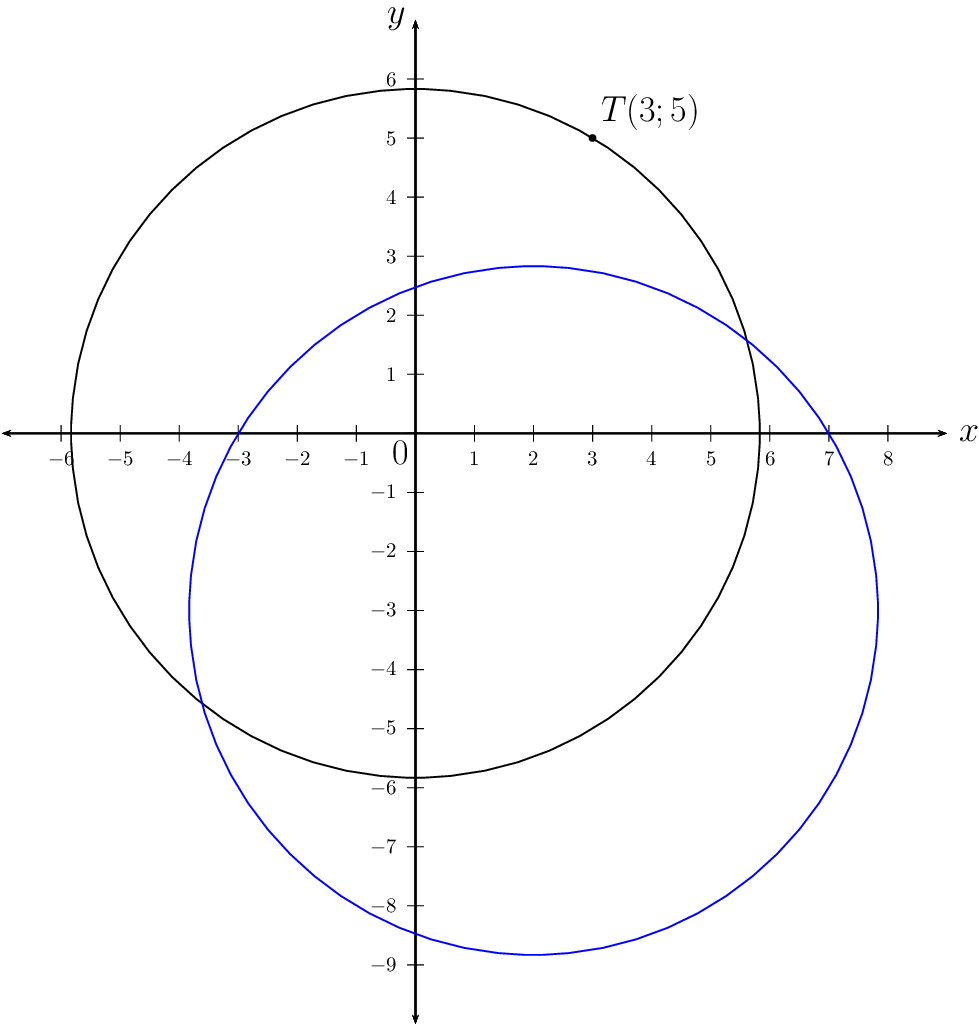On the same system of axes as the previous question, draw a sketch of the shifted circle reflected about the $$x$$-axis. State the coordinates of the centre of this circle.Centre of the shifted circle: $$(2;3)$$

Determine whether the circle $$x^{2} - 4x + y^{2} - 6y + 9 = 0$$ cuts, touches or does not intersect the $$x$$-axis and the $$y$$-axis.

\begin{align*} x^{2} - 4x + y^{2} - 6y + 9 &= 0 \\ (x - 2)^{2} - 4 + (y - 3)^{2} - 9 &= -9 \\ (x - 2)^{2} + (y - 3)^{2} &= 4 \\ \therefore (x - 2)^{2} + (y - 3)^{2} &= 4 \end{align*}

The radius of the circle is $$\text{2}$$ units. The distance from the centre to the $$y$$-axis is $$\text{2}$$ units, therefore the circle will touch the $$y$$-axis. The distance from the centre to the $$x$$-axis is $$\text{3}$$ units, therefore the circle will not intersect with the $$x$$-axis.